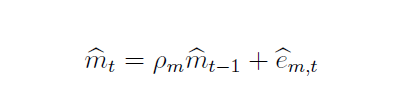# How to decide size of shock to effect a variable by a certain percentage?

Hi Everybody,

I am working with a log-linearized RBC model. It has a time-varying Loan-to-Value (LTV) shock to a borrowing constraint. It readswhere m is steady-state LTV ratio and eps_{m,t} is a shock with mean one. The log-linearized process for LTV isI want to do a simulation in which I fix size of LTV ratio to, let’s say, 0.95 and then hit it with a shock (in this case, eps_{m,t}) that reduces it by a percentage point (from 0.95 to 0.94). How do I do it? Since my model is log-linearized, all variables in steady-state are zero. As of now, I set the steady-state value of m as 0.95 as a parameter and then specify law of motion of \hat{m}_{t} in the model block. When I run stock_simul, I get steady state values of all variables as 0 and I don’t know how to set size of shock or how to see if LTV ratio goes from 0.95 to 0.94 on impact. Will appreciate any help.

If the model is log-linearized, everything is in percentage deviations from steady state. If you want m_t to decrease by 0.01 units, then the shock needs to be 0.01/0.95=0.0105 percent.

1 Like